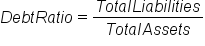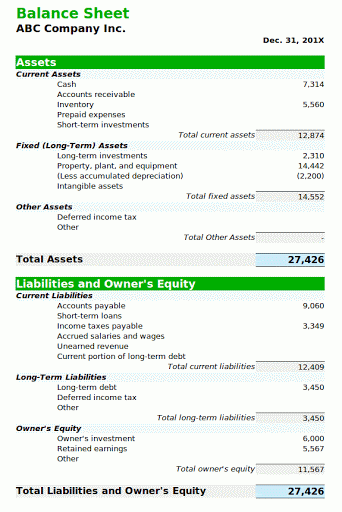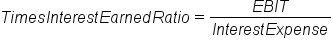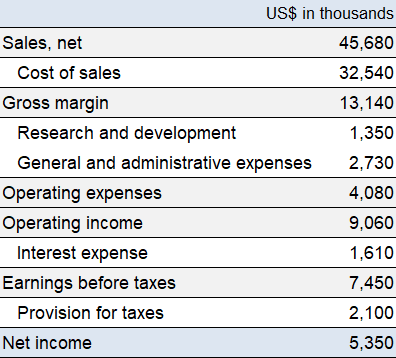+

# Debt Management Ratios

Author: Sophia Tutorial
##### Description:

Interpret the ratios that determine how effectively a company can handle its debt.

(more)### Developing Effective Teams

*No strings attached. This college course is 100% free and is worth 1 semester credit.

37 Sophia partners guarantee credit transfer.

299 Institutions have accepted or given pre-approval for credit transfer.

* The American Council on Education's College Credit Recommendation Service (ACE Credit®) has evaluated and recommended college credit for 32 of Sophia’s online courses. Many different colleges and universities consider ACE CREDIT recommendations in determining the applicability to their course and degree programs.

Tutorial

what's covered
In this lesson, you will learn about ratios that determine how effectively a company can handle its debt. Specifically, this lesson will cover:
1. Debt Ratio
2. Times Interest Earned Ratio

## 1. Debt Ratio

Debt management ratios measure a firm's ability to repay its long-term debt. Two ratios that will be discussed today are:

• Debt ratio
• Times interest earned ratio.
The debt ratio is a financial ratio that indicates the percentage of a company's assets that are provided by debt. It is the ratio of the total debt, which is the sum of the current liabilities and the long-term liabilities, divided by the total assets.

formula

Debt RatioThe higher this ratio, the greater the risk is with the firm's operations. Additionally, a high debt ratio may indicate a low borrowing capacity of a firm. This, in turn, will lower the firm's financial flexibility. Like all the other ratios, a debt ratio should be compared with the industry average, or with their competition.

### IN CONTEXT

Let's take a look at the balance sheet for the ABC Company.The total assets are \$27,426. The total liabilities are \$12,409 for the current liabilities and \$3,450 for the long-term liabilities, for a total of \$15,859. If we divide the total liabilities by the total assets, we get 0.58. This means that 58% of ABC's assets are financed by debt rather than by equity. Upon comparison, we may find that this is high and may limit their financial flexibility and borrowing power.
term to know

Debt Ratio
A ratio that indicates the percentage of a company's assets that are provided by debt.

## 2. Times Interest Earned Ratio

A second debt management ratio is the times interest earned ratio. The times interest earned, or the TIE, measures the company's ability to honor its debt payments. This is also sometimes called interest coverage.

It is calculated by dividing the EBIT, earnings before interest and taxes, by the interest charges.

formula

Times Interest Earned RatioIt is a great tool for measuring a company's ability to meet its debt obligations. Typically, it's a warning sign when the interest coverage falls below 2.5. When the times interest earned is less than one, the company is not generating enough cash from operations to meet its interest obligations. The company would either have to use cash on hand to make up the difference or borrow funds.

### IN CONTEXT

Let's take a look at another fictitious company.In this simple example, we are looking for the earnings before interest and taxes. That number here is the operating income of \$9,060,000, and the interest expense is \$1,610,000. If we divide \$9,060,000 by \$1,610,000, we get 5.62. So, in this example, this company is in fairly good shape in terms of interest coverage.
term to know

Times Interest Earned Ratio
A ratio that measures the company's ability to honor its debt payments.
summary
In this lesson, you learned about the debt ratio, which measures a firm's ability to repay its long-term debt by indicating what percentage of the company's assets are provided via debt, calculated by total liabilities divided by total assets. The higher the ratio, the greater the risk associated with the operation. The times interest earned ratio, or T.I.E., is a measure of a company's ability to honor its debt payments. It can be calculated as earnings before interest and taxes, divided by the total interest payable. Times interest earned, also called interest coverage, is a great tool to use when measuring a company's ability to meet its obligations

Best of luck in your learning!

Source: THIS CONTENT HAS BEEN ADAPTED FROM LUMEN LEARNING'S “DEBT MANAGEMENT RATIOS” TUTORIAL.

Terms to Know
Debt Ratio

A ratio that indicates the percentage of a company's assets that are provided by debt.

Times Interest Earned Ratio

A ratio that measures the company's ability to honor its debt payments.

Formulas to Know
Debt RatioTimes Interest Earned RatioRating